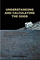# Statistics: Dice Problems

A few fun statistic problems with 6 sided dice

Given a six sided die the chances of you guessing 1 of those 6 different numbers {1,2,3,4,5,6} is 1 out of 6 or 1/6.

The chances that we have given a six sided die roll of guessing the number it landed on is 1/6, the values on that die are {1,2,3,4,5,6}.

The number of even numbers {2,4,6} on the die is 3.
The number of odd numbers (1,3,5} on the die is 3.

The question is basically asking what’s the chances of guessing one of the three even numbers that the die could possibly roll on, and the answer is 1 out of 3 or 1/3.

P(roll an even value) = 3/6 = 1/2
P(roll an odd value) = 3/6 = 1/2

Let’s take a look at some of the possible rolls
P(roll an even value) and P(roll an odd value) = (1/2) (1/2) = 1/4
P(roll an odd value)and P(roll an even value) = (1/2) (1/2) = 1/4
P(roll an odd value) and P(roll an odd value) = (1/2) (1/2) = 1/4
P(roll an even value) and P(roll an even value) = (1/2) (1/2) = 1/4

Now out of those 4 possibilities, 3 of them contain at least 1 odd roll. So our answer is 1/4 + 1/4 + 1/4 = 3/4

or

equivalently one minus the chances of getting two even rolls = 1 — (1/4) = 3/4.

Suppose we roll two dice. It is assume each die is fair and 6-sided. It means a roll of any value, the probability equal 1/6. Each die has 3 even values {2,4,6} and 3 odd values {1,3,5}.

NOTE: The following sums
(i) even + even = even
(ii) even + odd = odd
(iii) odd + odd = even
(iv) odd + even = odd

This means that we need one die to be odd and the other to be even in order for the sum of the two dice to be odd. So we want to know the probability of getting (odd and even) or (even and odd).

That probability is calculated below:
P(odd) = Probability of getting an odd number
P(even) = Probability of getting an even number

P(odd) and P(even) = (3/6)(3/6) = (1/2)(1/2) = (1/4)
P(even) and P(odd) = (3/6)(3/6) = (1/2)(1/2) = (1/4)

Probability of getting an (even and odd) or (odd and even):
= P( ( P(odd) and P(even) ) or (P(even) and P(odd)) )
= (1/4) + (1/4) = (2/4) = (1/2)

# Books For Extra Learning

Understanding and Calculating the Odds: Probability Theory Basics and Calculus Guide for Beginners, with Applications in Games of Chance and Everyday Life is a great book for more problems like the ones in this article and can help you beat the odds ! It goes from a basic introduction of probability to theory, combinatorics, and beginners calculus, all of which can be applied to games of chance.Understanding and Calculating the Odds: Probability Theory Basics and Calculus Guide for Beginners, with Applications in Games of Chance and Everyday Life

Another great book on statistics is Introduction to Statistical Learning with applications in R. An Introduction to Statistical Learning provides an accessible overview of the field of statistical learning, an essential tool set for making sense of the vast and complex data sets that have emerged in fields ranging from biology to finance to marketing to astrophysics in the past twenty years. This book presents some of the most important modeling and prediction techniques, along with relevant applications. Topics include linear regression, classification, re-sampling methods, shrinkage approaches, tree-based methods, support vector machines, clustering, and more. Color graphics and real-world examples are used to illustrate the methods presented. Since the goal of this textbook is to facilitate the use of these statistical learning techniques by practitioners in science, industry, and other fields, each chapter contains a tutorial on implementing the analyses and methods presented in R, an extremely popular open source statistical software platform.

# Check Out the following for content / videos on Computer Science, Algorithm Analysis, Programming, Mathematics and Logic:

compsci112358:

Video Tutorials on Recurrence Relation:

Video Tutorial on Algorithm Analysis:
https://www.udemy.com/algorithm-analysis/